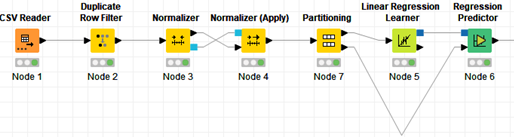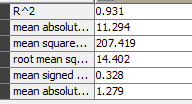# Performance expectation, Future performance of my algorithm

Hi everyone!
I’m learning regression and trying regression datasets.I built following simple modelNow I need to estimate the future performance of algorithm (Question 1). How do I do that in Knime.?
Also what would be performance expectation (Question 2)? How can I be sure that the estimation is close to true performance(Question 3).?

Hi,
Question 1:you can use the scorer node on the data you reserved on the second port to test.
Question 2: The statistics you find as output on the scorer node answers this question.
Question 3: If the data you used to train/test is a random sample of the data you’ll be using your model on in general it should perform similarly. Analyze the results and try to be sure you are not overfitting

1 Like

thanks iperez!

I don’t quite get your point. This is what I get after running the workflow.How can I tell the future performance ? by using R^2 ? Did I understand correctly that R^2 is the answer ?

R² is a measure of the degree of fitness of your model to your data. It measures the percentage of the variation of y explained by your model.
The mean absolute error is the average of the absolute value of the differences between real and forecasted values.

Chapter 3 of https://www.statlearning.com/ explains the theory behind and should help you to interpret the output of the node

2 Likes

Sorry for asking more. I don’t have access to this book. Can you please explain in simple terms how can I answer all these by using my simple model?
Thanks!

2 Likes

There is no need to use Normalizer Apply after the Normalizer node. The apply is for your test set.

1 Like

Sorry. Can you elaborate it a little bit? Thanks

Thanks dude. Let me read. I will post my findings.

Here’s a recent blog post from our Evangelism team covering much the same ground:

https://towardsdatascience.com/numeric-scoring-metrics-acd3896c5eff

2 Likes

This topic was automatically closed 7 days after the last reply. New replies are no longer allowed.